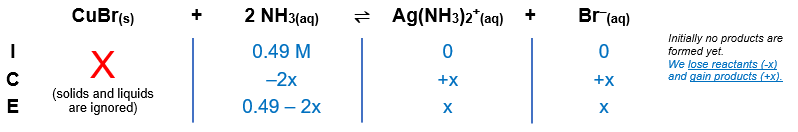# Problem: Copper(I) ions in aqueous solution react with NH 3(aq) according to  Cu+ (aq) + 2NH3 (aq) → Cu(NH3)2 + (aq)      Kf = 6.3 x 1010 Calculate the solubility (in g.L-1) of CuBr(s) (Ksp = 6.3 x 10-9) in 0.49 M NH 3 (aq).

###### FREE Expert Solution

Cu+(aq) + 2 NH3(aq) → Cu(NH3)2+(aq)                Kf = 6.3×1010
CuBr(s) → Cu+(aq) + Br(aq)                                 Ksp = 6.3×10‒9
_____________________________________________________
CuBr(s) + 2 NH3(aq) → Cu(NH3)2+(aq) + Br(aq)            K = Kf × Ksp

$\mathbf{K}\mathbf{=}{\mathbf{K}}_{\mathbf{f}}\mathbf{×}{\mathbf{K}}_{\mathbf{sp}}\phantom{\rule{0ex}{0ex}}\mathbf{K}\mathbf{=}\left(6.3×{10}^{10}\right)\left(6.3×{10}^{-9}\right)$

K = 396.9We can now plug in the equilibrium concentrations and solve for x, which is the molar solubility of CuBr in M or mol/L.

86% (385 ratings)###### Problem Details

Copper(I) ions in aqueous solution react with NH 3(aq) according to

Cu+ (aq) + 2NH3 (aq) → Cu(NH3)+ (aq)      K= 6.3 x 1010

Calculate the solubility (in g.L-1) of CuBr(s) (Ksp = 6.3 x 10-9) in 0.49 M NH (aq).